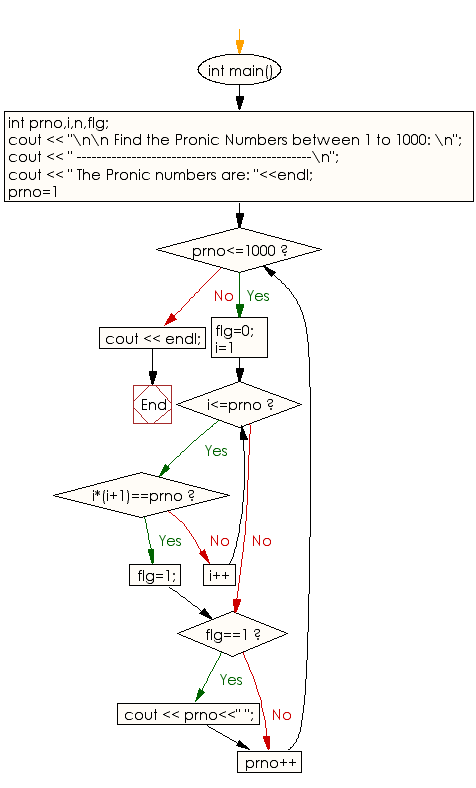﻿ C++ Exercises: Find Pronic Number between 1 to 1000 - w3resource

# C++ Exercises: Find Pronic Number between 1 to 1000

## C++ Numbers: Exercise-23 with Solution

Write a program in C++ to find Pronic numbers between 1 and 1000.

Sample Solution:

C++ Code :

``````#include<bits/stdc++.h>
using namespace std;

int main()
{
int prno,i,n,flg;
cout << "\n\n Find the Pronic Numbers between 1 to 1000: \n";
cout << " -----------------------------------------------\n";
cout << " The Pronic numbers are: "<<endl;
for(prno=1;prno<=1000;prno++)
{
flg=0;
for(i=1;i<=prno;i++)
{
if(i*(i+1)==prno)
{
flg=1;
break;
}
}
if(flg==1)
{
cout << prno<<" ";
}
}
cout << endl;
}
``````

Sample Output:

``` Find the Pronic Numbers between 1 to 1000:
-----------------------------------------------
The Pronic numbers are:
2 6 12 20 30 42 56 72 90 110 132 156 182 210 240 272 306 342 380 420 462 506 552 600 650 702 756 812
870 930 992
```

Flowchart:C++ Code Editor: